Home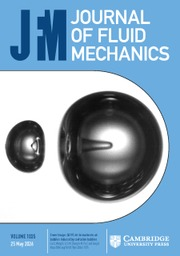Journal of Fluid Mechanics

# Turbulent thermal convection over rough plates with varying roughness geometries

Published online by Cambridge University Press:  21 July 2017

Corresponding

## Abstract

We present a systematic investigation of the effects of roughness geometry on turbulent Rayleigh–Bénard convection (RBC) over rough plates with pyramid-shaped and periodically distributed roughness elements. Using a parameter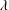$\unicode[STIX]{x1D706}$ defined as the height of a roughness element over its base width, the heat transport, the flow dynamics and the local temperatures are measured for the Rayleigh number range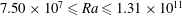$7.50\times 10^{7}\leqslant Ra\leqslant 1.31\times 10^{11}$ and Prandtl numbers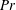$Pr$ from 3.57 to 23.34 at four values of$\unicode[STIX]{x1D706}$ (0.5, 1.0, 1.9 and 4.0). It is found that the heat transport scaling, i.e.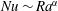$Nu\sim Ra^{\unicode[STIX]{x1D6FC}}$ where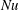$Nu$ is the Nusselt number, may be classified into three regimes in turbulent RBC over rough plates. In Regime I, the system is in a dynamically smooth state. The heat transport scaling is the same as that in a smooth cell. In Regimes II and III, the heat transport is enhanced. When$\unicode[STIX]{x1D706}$ is increased from 0.5 to 4.0,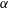$\unicode[STIX]{x1D6FC}$ increases from 0.36 to 0.59 in Regime II and it increases from 0.30 to 0.50 in Regime III. The experiment thus clearly demonstrates that the heat transport scaling in turbulent RBC can be manipulated using$\unicode[STIX]{x1D706}$ in the heat transport enhanced regime. Previous studies suggest that the transition to heat transport enhanced regime, i.e. from Regime I to Regime II, occurs when the thermal boundary layer (BL) thickness becomes smaller than the roughness height. Direct measurements of the viscous BL in the present study suggest that the transition from Regime II to Regime III is likely a result of the viscous BL thickness becoming smaller than the roughness height. The scaling exponent of the Reynolds number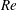$Re$ with respect to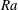$Ra$ changes from 0.471 to 0.551 when$\unicode[STIX]{x1D706}$ is increased from 0.5 to 4.0, suggesting a change of the dynamics of the large-scale circulation. Interestingly, the transition from Regime II to Regime III in terms of the heat transport scaling is not reflected in the$Re$ scaling with$Ra$ . It is also found that increasing$\unicode[STIX]{x1D706}$ increases the clustering of thermal plumes which effectively increases the plume lifetime. This leads to a great increase in the probability of observing large temperature fluctuations in the bulk flow, which corresponds to the formation of more coherent plumes or plume clusters that are ultimately responsible for the enhanced heat transport.

## JFM classification

Type
Papers
Information
Journal of Fluid Mechanics , 25 August 2017 , pp. 573 - 599

## Access options

Get access to the full version of this content by using one of the access options below.

## References

Ahlers, G., Grossmann, S. & Lohse, D. 2009 Heat transfer and large-scale dynamics in turbulent Rayleigh–Bénard convection. Rev. Mod. Phys. 81, 503537.CrossRefGoogle Scholar
Brown, E., Nikolaenko, A.i, Funfschilling, D. & Ahlers, G. 2005 Heat transport in turbulent Rayleigh–Bénard convection: effect of finite top- and bottom-plate conductivities. Phys. Fluids 17, 075108.CrossRefGoogle Scholar
Chillá, F. & Schumacher, J. 2012 New perspectives in turbulent Rayleigh–Bénard convection. Euro. Phys. J. E 35, 125.Google ScholarPubMed
Ciliberto, S. & Laroche, C. 1999 Random roughness of boundary increases the turbulent convection scaling exponent. Phys. Rev. Lett. 82, 39984001.CrossRefGoogle Scholar
Du, Y.-B. & Tong, P. 2000 Turbulent thermal convection in a cell with ordered rough boundaries. J. Fluid Mech. 407, 5784.CrossRefGoogle Scholar
Du, Y.-B. & Tong, P. 2001 Temperature fluctuations in a convection cell with rough upper and lower surfaces. Phys. Rev. E 63, 046303.Google Scholar
Du, Y.-B., Tong, P. & Xia, K.-Q. 1998 Enhanced heat transport in turbulent convection over a rough surface. Phys. Rev. Lett. 81, 987990.CrossRefGoogle Scholar
Funfschilling, D. & Ahlers, G. 2004 Plume motion and large-scale circulation in a cylindrical Rayleigh–Bénard cell. Phys. Rev. Lett. 92, 194502.CrossRefGoogle Scholar
Funfschilling, D., Brown, E., Nikolaenko, A. & Ahlers, G. 2005 Heat transport by turbulent Rayleigh–Bénard convection in cylindrical samples with aspect ratio one and larger. J. Fluid Mech. 536, 145154.CrossRefGoogle Scholar
Goluskin, David & Doering, C. R. 2016 Bounds for convection between rough boundaries. J. Fluid Mech. 804, 370386.CrossRefGoogle Scholar
Grossmann, S. & Lohse, D. 2000 Scaling in thermal convection: a unifying theory. J. Fluid Mech. 407, 2756.CrossRefGoogle Scholar
Grossmann, S. & Lohse, D. 2001 Thermal convection for large Prandtl numbers. Phys. Rev. Lett. 86, 33163319.CrossRefGoogle ScholarPubMed
Grossmann, S. & Lohse, D. 2002 Prandtl and Rayleigh number dependence of the Reynolds number in turbulent thermal convection. Phys. Rev. E 66, 016305.Google ScholarPubMed
Grossmann, S. & Lohse, D. 2004 Fluctuations in turbulent Rayleigh–Bénard convection: The role of plumes. Phys. Fluids 16, 44624472.CrossRefGoogle Scholar
Kraichnan, R. H. 1962 Turbulent thermal convection at arbitrary Prandtl number. Phys. Fluids 5, 1374.CrossRefGoogle Scholar
Lam, S., Shang, X.-D., Zhou, S.-Q. & Xia, K.-Q. 2002 Prandtl number dependence of the viscous boundary layer and the Reynolds numbers in Rayleigh–Bénard convection. Phys. Rev. E 65, 066306.Google ScholarPubMed
Liot, O., Salort, J., Kaiser, R., du Puits, R. & Chillá, F. 2016 Boundary layer structure in a rough Rayleigh–Bénard cell filled with air. J. Fluid Mech. 786, 275293.CrossRefGoogle Scholar
Lohse, D. & Xia, K.-Q. 2010 Small-scale properties of turbulent Rayleigh–Bénard convection. Annu. Rev. Fluid Mech. 42, 335364.CrossRefGoogle Scholar
Qiu, X.-L. & Xia, K.-Q. 1998 Viscous boundary layers at the sidewall of a convection cell. Phys. Rev. E 58, 486491.Google Scholar
Qiu, X.-L., Xia, K.-Q. & Tong, P. 2005 Experimental study of velocity boundary layer near a rough conducting surface in turbulent natural convection. J. Turbul. 6, N30.CrossRefGoogle Scholar
Roche, P. E., Castaing, B., Chabaud, B. & Hébral, B. 2001 Observation of the 1/2 power law in Rayleigh–Bénard convection. Phys. Rev. E 63, 045303.Google Scholar
Shen, Y., Tong, P. & Xia, K.-Q. 1996 Turbulent convection over rough surfaces. Phys. Rev. Lett. 76, 908911.CrossRefGoogle ScholarPubMed
Shishkina, O. & Wagner, C. 2011 Modelling the influence of wall roughness on heat transfer in thermal convection. J. Fluid Mech. 686, 568582.CrossRefGoogle Scholar
Siggia, E. D. 1994 High Rayleigh number convection. Annu. Rev. Fluid Mech. 26, 137168.CrossRefGoogle Scholar
Stringano, G., Pascazio, G. & Verzicco, R. 2006 Turbulent thermal convection over grooved plates. J. Fluid Mech. 557, 307336.CrossRefGoogle Scholar
Sun, C., Cheung, Y.-H. & Xia, K.-Q. 2008 Experimental studies of the viscous boundary layer properties in turbulent Rayleigh–Bénard convection. J. Fluid Mech. 605, 79113.CrossRefGoogle Scholar
Sun, C., Ren, L.-Y., Song, H. & Xia, K.-Q. 2005 Heat transport by turbulent Rayleigh–Bénard convection in 1 m diameter cylindrical cells of widely varying aspect ratio. J. Fluid Mech. 542, 165174.CrossRefGoogle Scholar
Tisserand, J. C., Creyssels, M., Gasteuil, Y., Pabiou, H., Gibert, M., Castaing, B. & Chillá, F. 2011 Comparison between rough and smooth plates within the same Rayleigh–Bénard cell. Phys. Fluids 23, 015105.CrossRefGoogle Scholar
Toppaladoddi, S., Succi, S. & Wettlaufer, J. S. 2015 Tailoring boundary geometry to optimize heat transport in turbulent convection. Europhys. Lett. 111, 44005.CrossRefGoogle Scholar
Toppaladoddi, S., Succi, S. & Wettlaufer, J. S. 2017 Roughness as a route to the ultimate regime of thermal convection. Phys. Rev. Lett. 118, 074503.CrossRefGoogle ScholarPubMed
Verzicco, R. 2004 Effects of nonperfect thermal sources in turbulent thermal convection. Phys. Fluids 16, 19651979.CrossRefGoogle Scholar
Wagner, S. & Shishkina, O. 2015 Heat flux enhancement by regular surface roughness in turbulent thermal convection. J. Fluid Mech. 763, 109135.CrossRefGoogle Scholar
Wei, P. & Ahlers, G. 2016 On the nature of fluctuations in turbulent Rayleigh–Bénard convection at large Prandtl numbers. J. Fluid Mech. 802, 203244.CrossRefGoogle Scholar
Wei, P., Chan, T.-S., Ni, R., Zhao, X.-Z. & Xia, K.-Q. 2014 Heat transport properties of plates with smooth and rough surfaces in turbulent thermal convection. J. Fluid Mech. 740, 2846.CrossRefGoogle Scholar
Wei, P., Ni, R. & Xia, K.-Q. 2012 Enhanced and reduced heat transport in turbulent thermal convection with polymer additives. Phys. Rev. E 86, 016325.Google ScholarPubMed
Wei, P. & Xia, K.-Q. 2013 Viscous boundary layer properties in turbulent thermal convection in a cylindrical cell: the effect of cell tilting. J. Fluid Mech. 720, 140168.CrossRefGoogle Scholar
Xi, H.-D., Zhou, S.-Q., Zhou, Q., Chan, T.-S. & Xia, K.-Q. 2009 Origin of the temperature oscillation in turbulent thermal convection. Phys. Rev. Lett. 102, 044503.CrossRefGoogle ScholarPubMed
Xia, K.-Q. 2013 Current trends and future directions in turbulent thermal convection. Theor. Appl. Mech. Lett. 3, 052001.CrossRefGoogle Scholar
Xia, K.-Q., Lam, S. & Zhou, S.-Q. 2002 Heat-flux measurement in high-Prandtl-number turbulent Rayleigh–Bénard convection. Phys. Rev. Lett. 88, 064501.CrossRefGoogle ScholarPubMed
Xia, K.-Q., Sun, C. & Zhou, S.-Q. 2003 Particle image velocimetry measurement of the velocity field in turbulent thermal convection. Phys. Rev. E 68, 066303.Google ScholarPubMed
Xie, Y.-C., Huang, S.-D., Funfschilling, D., Li, X.-M., Ni, R. & Xia, K.-Q. 2015 Effects of polymer additives in the bulk of turbulent thermal convection. J. Fluid Mech. 784, R1.CrossRefGoogle Scholar
Xie, Y.-C., Wei, P. & Xia, K.-Q. 2013 Dynamics of the large-scale circulation in high-Prandtl-number turbulent thermal convection. J. Fluid Mech. 717, 322346.CrossRefGoogle Scholar
Xin, Y.-B. & Xia, K.-Q. 1997 Boundary layer length scales in convective turbulence. Phys. Rev. E 56, 30103015.Google Scholar
Xin, Y.-B., Xia, K.-Q. & Tong, P. 1996 Measured velocity boundary layers in turbulent convection. Phys. Rev. Lett. 77, 12661269.CrossRefGoogle ScholarPubMed
Zhou, S.-Q. & Xia, K.-Q. 2001 Scaling properties of the temperature field in convective turbulence. Phys. Rev. Lett. 87, 064501.CrossRefGoogle ScholarPubMed
Zhou, S.-Q., Xie, Y.-C., Sun, C. & Xia, K.-Q. 2016 Statistical characterization of thermal plumes in turbulent thermal convection. Phys. Rev. Fluids 1, 054301.CrossRefGoogle Scholar
Zhu, X., Ostilla-Mónico, R., Verzicco, R. & Lohse, D. 2016 Direct numerical simulation of Taylor–Couette flow with grooved walls: torque scaling and flow structure. J. Fluid Mech. 794, 746774.CrossRefGoogle Scholar

### Full text views

Full text views reflects PDF downloads, PDFs sent to Google Drive, Dropbox and Kindle and HTML full text views.

Total number of HTML views: 31
Total number of PDF views: 409 *
View data table for this chart

* Views captured on Cambridge Core between 21st July 2017 - 27th January 2021. This data will be updated every 24 hours.

Hostname: page-component-898fc554b-8ljtz Total loading time: 0.573 Render date: 2021-01-27T23:28:23.663Z Query parameters: { "hasAccess": "0", "openAccess": "0", "isLogged": "0", "lang": "en" } Feature Flags: { "shouldUseShareProductTool": true, "shouldUseHypothesis": true, "isUnsiloEnabled": true, "metricsAbstractViews": false, "figures": false, "newCiteModal": false }

# Send article to Kindle

Note you can select to send to either the @free.kindle.com or @kindle.com variations. ‘@free.kindle.com’ emails are free but can only be sent to your device when it is connected to wi-fi. ‘@kindle.com’ emails can be delivered even when you are not connected to wi-fi, but note that service fees apply.

Find out more about the Kindle Personal Document Service.

Turbulent thermal convection over rough plates with varying roughness geometries
Available formats
×

# Send article to Dropbox

To send this article to your Dropbox account, please select one or more formats and confirm that you agree to abide by our usage policies. If this is the first time you use this feature, you will be asked to authorise Cambridge Core to connect with your <service> account. Find out more about sending content to Dropbox.

Turbulent thermal convection over rough plates with varying roughness geometries
Available formats
×

# Send article to Google Drive

To send this article to your Google Drive account, please select one or more formats and confirm that you agree to abide by our usage policies. If this is the first time you use this feature, you will be asked to authorise Cambridge Core to connect with your <service> account. Find out more about sending content to Google Drive.

Turbulent thermal convection over rough plates with varying roughness geometries
Available formats
×
×